## 12/29/2011

### (TIP) The method to convert 4 bytes array to float

If you have 4 byte array, for example buffer, you can change the byte buffer to float.

ex)

```union BitConvert{
float f;
unsigned long ul;
};

unsigned char a,b,c,d;
a = buffer;
b = buffer;
c = buffer;
d = buffer;

BitConvert EX;
EX.ul = (a << 24)  | (b << 16) | (c << 8) | d;
// or EX.ul = (d << 24) | ( c << 16)  | (b << 8) | a; (It is depend on your plaform.)
```

There is float value in the "EX.f".

Thank you~. ^^

### (TIP) The mean of CR, LF in the serial communication

The mean of the , is like that... in the serial communication.

LF = 10 = 0x0A = '\n'
CR = 13 = 0x0d = '\r'

Thanks you. ^^

## 12/26/2011

### How to install OpenGL in Window OS and Visula C++ 2008

The Zip file has below files.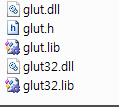1) You have to copy these file into right directories.

x86  (32bit)
glut32.dll   = C:\Windows\System32
glut.dll       = C:\Windows\System32

x64 (62bit)
glut32.dll   = C:\Windows\sysWOW64
glut.dll       = C:\Windows\sysWOW64

glut.h        = C:\Program Files\Microsoft SDKs\Windows\v6.0A\Include\gl

glut.lib      = C:\Program Files\Microsoft SDKs\Windows\v6.0A\Lib
glut32.lib = C:\Program Files\Microsoft SDKs\Windows\v6.0A\Lib

※  If you met some errors, Copy files additional folder.
C:\Program Files\Microsoft Visual Studio 9.0\VC\lib
C:\Program Files\Microsoft Visual Studio 9.0\VC\include
copy glut.lib, glut32.lib and glut.h into additional folders.

2) Visual Studio Setting

This is example source code.

```#include
void Draw()
{
glClear(GL_COLOR_BUFFER_BIT);

glColor3f(1.0f, 0.0f, 1.0f);
glVertex3f(-0.5f, 0.5f, 0.5f);
glVertex3f(0.5f, 0.5f, 0.5f);
glVertex3f(0.5f, -0.5f, 0.5f);
glVertex3f(-0.5f, -0.5f, 0.1f);
glEnd();

glFlush();
}

void main()
{
glutCreateWindow("NeMo");
glutDisplayFunc(Draw);
glutMainLoop();
}
```

This setting process is normal course, but I met the this errors message in the Visual Studio 2008.
1>NeMo.obj : error LNK2001: __imp____glutCreateWindowWithExit@8 외부 기호를 확인할 수 없습니다.
1>C:\Users\mare\Documents\네이트온 받은 파일\NeMo\Debug\NeMo.exe : fatal error LNK1120: 1개의 확인할 수 없는 외부 참조입니다.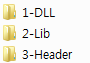I have done directory setting in the Visual Studio options.
And you have to change #include <~> to #include "~" on the source code.

I want to suceess to complie OpneGL Project on your machine.
Thanks you.

## 11/29/2011

### (TIP) Matlab Plot Option

colormap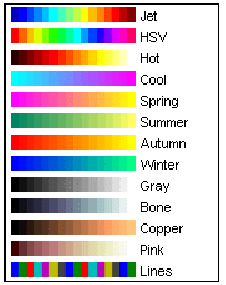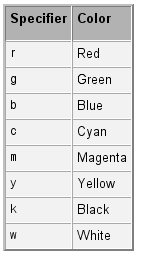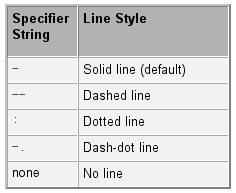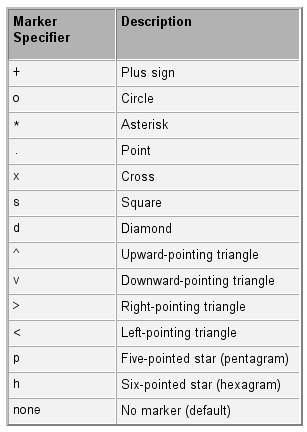Below code is example of matlab help document.
x = -pi:pi/10:pi;
y = tan(sin(x)) - sin(tan(x));
plot(x,y,'--rs','LineWidth',2,...
'MarkerEdgeColor','k',...
'MarkerFaceColor','g',...
'MarkerSize',10)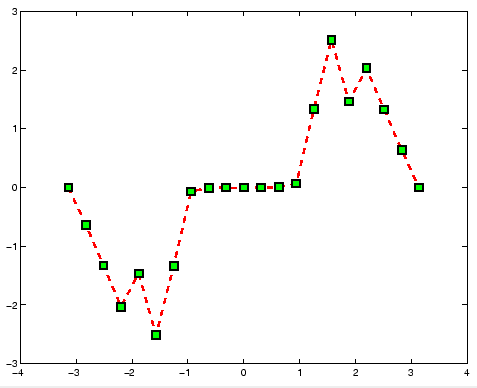You can describe axes on your image.
figure(1);
iptsetpref('ImshowAxesVisible','on');
imshow(data2); %'colormap',bone(0)
set(gca, 'XTickLabel', {'a', 'b', 'c', 'd', 'e', 'f'} );
set(gca, 'YTickLabel', {100:100:700} );
xlabel('Longitude');ylabel('Latitude');

## 11/27/2011

### (TIP) Making a file name appending number in Matlab

It is simple and easy but I can not remember the code well when I need this code.
So I leave this code in my blog.
And I hope the code is also useful to all visitor.
Thank you.

endIndex = 10;
for j=1:endIndex
Index = num2str(j);
end

->
This source code can load below file names sequentially.
1saveFile.txt
2saveFile.txt
3saveFile.txt
4saveFile.txt
5saveFile.txt
6saveFile.txt
7saveFile.txt
...

## 11/25/2011

### Screen capture and save program/ C++ source code

Created Date : 2011.11
Language :C++
Tool : Visual Studio 2008
Library & Utilized : CImage
Reference : Internet
etc. : -

I made this program to help my friend.
This progrma is screen capture program.
At first, we do whole screen capture and set region of screen to capture.
Then start capture and image saving in real-time.
The image file is saved in your program directory.
The file name of the saved image is like that "Time.jpg(EX: 11223242.jpg, 11223243.jpg...)"

The program is made based on below capture program.

```#include
void Capture()
{

int nx =0, ny = 0;
CImage capImage;
CWnd *pDesktopWnd = GetDesktopWindow();
HDC hDC = NULL;
if(!pDesktopWnd)
return;
CWindowDC DeskTopDC(pDesktopWnd);
nx = GetSystemMetrics(SM_CXSCREEN);
ny = GetSystemMetrics(SM_CYSCREEN);
if(!capImage.Create(nx, ny, 32))
return;
hDC = capImage.GetDC();
BitBlt(hDC, 0,0, nx, ny, DeskTopDC.m_hDC, 0, 0, SRCCOPY);
capImage.Save(_T("test.jpg"), Gdiplus::ImageFormatJPEG);
capImage.ReleaseDC();

}
```

I am hard to make file name of capture image because the progrma requires UNICODE type character.

So I solved this problem as below code.

```    int T = time(NULL);
TCHAR str;
wsprintf(str,_T("%d.jpg"),T);
ImageTemp.Save(str, Gdiplus::ImageFormatJPEG);
ImageTemp.ReleaseDC();
```

source code

## 11/16/2011

### Thread Test in the C++ console mode.

The goal of this source code is how to send large data to the thread and how to wait until all thread finish their job.

I use struct to send the large data.
and I use the flags for waiting the time of the all thread finish.

<source code>

```//////////////////////////////////////////////////////////////////////////
// Made by J.H.KIM, 2011 / feelmare@daum.net, feelmare@gmail.com        //
// blog : http://feelmare.blogspot.com                                  //
// My Lab : VISLAB(http://me.pusan.ac.kr)                               //
//////////////////////////////////////////////////////////////////////////

#include <stdio.h>
#include <process.h>
#include <windows.h>

struct InputData
{
int * data;
int width;
int height;
int OriginW;
int reserved;
int flag;
};

void main()
{

//Data Allocation and Setting
int W = 100;
int H = 100;
int * buffer;
buffer =new int[W*H];

for(int i=0; i<W*H; ++i)
{
buffer[i] = i;
}

int sx,sy,w,h;

InputData th1_input;
sx = 0; sy = 0; w = 80; h = 90; //(80x90) image processing
th1_input.width = w;
th1_input.height = h;
th1_input.OriginW = W;
th1_input.data = &buffer[sy*W+sx];
th1_input.reserved = 1;
th1_input.flag = TRUE;

InputData th2_input;
sx = 10; sy = 10; w = 50; h = 50; //(50x50) image processing
th2_input.width = w;
th2_input.height = h;
th2_input.OriginW = W;
th2_input.data = &buffer[sy*W+sx];
th2_input.reserved = 2;
th2_input.flag = TRUE;

InputData th3_input;
sx = 10; sy = 10; w = 20; h = 20; //(10x10) image processing
th3_input.width = w;
th3_input.height = h;
th3_input.OriginW = W;
th3_input.data = &buffer[sy*W+sx];
th3_input.reserved = 3;
th3_input.flag = TRUE;

//wait until all thread is finished.
while( th1_input.flag || th2_input.flag || th3_input.flag)
{
Sleep(100);
}

//Data buffer release
delete[] buffer;

}

//copy struct data to variable
int w = ((InputData*)pParam)->width;
int h = ((InputData*)pParam)->height;
int ow = ((InputData*)pParam)->OriginW;

//Processing Sum(v^2.)
int v;
double sum=0;
for(int j=0; j<h; ++j)
{
for(int i=0; i<w; ++i)
{
v = ((InputData*)pParam)->data[j*ow+i];
sum += (v*v);
}
}

printf("%d thread value = %lf\n", ((InputData*)pParam)->reserved , sum);

((InputData*)pParam)->flag = FALSE;
}

```

Please leave comment if the source code has problem.
Thank you~

## 11/15/2011

### Web Cam Capture source code using OpenCV

This source code is very simple. But the code will be help to somebody who start to study the opencv.
Thank you~.

```    //image class
IplImage* image = 0;
//camera point
CvCapture* capture = cvCaptureFromCAM(0);
//make window
cvNamedWindow( "camera");

//image class for processing
IplImage* image2=0;
//make window
cvNamedWindow( "camera2");

unsigned char R,G,B;
while(1) {
//capture a frame form cam
cvGrabFrame( capture );
//copy to image class
image = cvRetrieveFrame( capture );

//create image at one time in the roof
if(image2 == 0)
{
image2  = cvCreateImage(cvSize(image->width, image->height), image->depth, image->nChannels);
}

//simple image processing
for(int i=0; i<image->height; ++i)
{
for(int j=0; j<image->width; ++j)
{
//to get R,G,B value
B = (unsigned char)image->imageData[i*image->widthStep+j*3+0];
G = (unsigned char)image->imageData[i*image->widthStep+j*3+1];
R = (unsigned char)image->imageData[i*image->widthStep+j*3+2];

//image color converting
B = 255-B;
G = 255-G;
R = 255-R;

//copy R,G,B value to image2
image2->imageData[i*image2->widthStep+j*3+0] = B;
image2->imageData[i*image2->widthStep+j*3+1] = G;
image2->imageData[i*image2->widthStep+j*3+2] = R;
}
}

//show window
cvShowImage( "camera", image );
cvShowImage( "camera2", image2 );

//break
if( cvWaitKey(10) >= 0 )
break;
}

//release imge
cvReleaseImage(&image2);
//release capture point
cvReleaseCapture( &capture );
//close the window
cvDestroyWindow( "camera" );
cvDestroyWindow( "camera2" );
```

## 11/11/2011

### Project - Embeded camera R&D for Real-Time fire detection surveillance in tunnel environment

Embeded camera R&D for Real-Time fire detection surveillance in tunnel environment
- 2008.07.01~2009.06.30
- High Computing Power Embeded Camera R&D, Fire & Smoke Detection(My Job)

The goal of the project is to develop embeded camera for detect fire and smoke.
My job was an algorithm R&D for detection fire & smoke and the s/w programing.
The fire detection algorithm uses the HMM algorithm.
The features of the fire's sequence is learned in the off-line. And then the learned Markov model is used in the oline using viterbi algorithm.

Below Movies are the result of the project.

*** Demo 1, Fire detection Simple situation :

*** Demo 2, Fire detection in the road, There is similar light of the car with fire color:

***Demo 3, Fire detection test in the real fire situation :

***Demo 4, Embeded Camera Test. The camera detects the fire and then zoom in the region of the fire.

***Demo 5, Smoke detection test.

## 11/08/2011

### 8 point algorithm (Matlab source code) / The method to get the Fundamental Matrix and the Essential matrix

Created Date : 2011.8
Language : Matlab
Tool : Matlab 2010
Library & Utilized : -
Reference : Multiple View Geometry (Hartly and Zisserman)
etc. : Intrinsic Parameter, 2 adjacent images, matching points

This code is 8 point algorithm.
If we know over 8 corresponding points between two images, we can know Rotation and Translation of camera movement using 8 point algorithm.
The 8 point algorithm is well known in the vision major field.
The algorihtm is introduced at the Multiple View Geometry Book and many websites.

Have you ever listened Fundamental matrix song? The song is very cheerful. ^^

My code is very simple. so I believe my code will be useful to you.
I will upload RANSAC version later.
Thank you.

github url :https://github.com/MareArts/8point-algorithm
(I used 'getCorrectCameraMatrix' function of Isaac Esteban author.)

main M code..
```%//////////////////////////////////////////////////////////////////////////
%// Made by J.H.KIM, 2011 / feelmare@daum.net, feelmare@gmail.com        //
%// blog : http://feelmare.blogspot.com                                  //
%// Eight-Point Algorithm
%//////////////////////////////////////////////////////////////////////////

clc; clear all; close all;

% Corresponding points between two images
% sample #1 I11.jpg, I22.jpg
%{
m1 = I11; m2 = I22;
%}

%sample #2 I1.jpg, I2.jpg
m1 = I1; m2 = I2;

s = length(m1);
m1=[m1(:,1) m1(:,2) ones(s,1)];
m2=[m2(:,1) m2(:,2) ones(s,1)];
Width = 800; %image width
Height = 600; %image height

% Intrinsic Matrix
K = intrinsic_matrix;

% The matrix for normalization(Centroid)
N=[2/Width 0 -1;
0 2/Height -1;
0   0   1];

%%
% Data Centroid
x1=N*m1'; x2=N*m2';
x1=[x1(1,:)' x1(2,:)'];
x2=[x2(1,:)' x2(2,:)'];

% Af=0
A=[x1(:,1).*x2(:,1) x1(:,2).*x2(:,1) x2(:,1) x1(:,1).*x2(:,2) x1(:,2).*x2(:,2) x2(:,2) x1(:,1) x1(:,2), ones(s,1)];

% Get F matrix
[U D V] = svd(A);
F=reshape(V(:,9), 3, 3)';
% make rank 2
[U D V] = svd(F);
F=U*diag([D(1,1) D(2,2) 0])*V';

% Denormalize
F = N'*F*N;
%Verification
%L1=F*m1'; m2(1,:)*L1(:,1); m2(2,:)*L1(:,2); m2(3,:)*L1(:,3);

%%
%Get E
E=K'*F*K;
% Multiple View Geometry 259page
%Get 4 Possible P matrix
P4 = get4possibleP(E);
%Get Correct P matrix
inX = [m1(1,:)' m2(1,:)'];
P1 = [eye(3) zeros(3,1)];
P2 = getCorrectCameraMatrix(P4, K, K, inX)

%%
%Get 3D Data using Direct Linear Transform(Linear Triangular method)
Xw = Triangulation(m1',K*P1, m2',K*P2);
xx=Xw(1,:);
yy=Xw(2,:);
zz=Xw(3,:);

figure(1);
plot3(xx, yy, zz, 'r+');

%{
%This code is also run well instead of Triangulation Function.
nm1=inv(K)*m1';
nm2=inv(K)*m2';
% Direct Linear Transform
for i=1:s
A=[P1(3,:).*nm1(1,i) - P1(1,:);
P1(3,:).*nm1(2,i) - P1(2,:);
P2(3,:).*nm2(1,i) - P2(1,:);
P2(3,:).*nm2(2,i) - P2(2,:)];

A(1,:) = A(1,:)./norm(A(1,:));
A(2,:) = A(2,:)./norm(A(2,:));
A(3,:) = A(3,:)./norm(A(3,:));
A(4,:) = A(4,:)./norm(A(4,:));

[U D V] = svd(A);
X(:,i) = V(:,4)./V(4,4);
end
%}

```

## 11/07/2011

### Rotation Matrix Converting Matlab Source (Euler Angle, Rotation Matrix, Quanternion)

There are many expression to show the rotation value.
(Ex. : Euler, Matrix, Quaternion.. )

This code is the test source to convert each other.
Euler -> Matrix -> Quanternion -> Matrix -> Euler
We can show the first Euler value is same with the last Euler value.

The source code is like below:
--------------------------------------------------------------

% Rotation vector of x,y,z axis.
Rv = [13 20 50];

% 3x3 matrix of R vector (Results of the Rm1 and Rm2 is similar.)
Rm1 = rodrigues(Rv*pi/180)
Rm2 = mRotMat(Rv)

% Quntenion vector of R matrix
Rq1 = matrix2quaternion(Rm1)
Rq2 = matrix2quaternion(Rm2)

% R matrix of Q vector
Rm1_1 = quaternion2matrix(Rq1)
Rm2_2 = quaternion2matrix(Rq1)

% R vector of R matrix
Rv_1 = rodrigues(Rm1_1(1:3,1:3)) * 180/pi
Rv_2 = rodrigues(Rm2_2(1:3,1:3)) * 180/pi

-----------------------------------------------------------------------
<Source Code>

The copyright of "rodrigues' and 'quaternion' functions is reserved by Peter Kovesi.

I wish this source code is useful to you.
Thank you.

## 11/04/2011

### A* path planning Algorithm / C++ source code

Created Date : 2010.8
Language : C/C++
Tool : Microsoft Visual C++ 6.0
Library & Utilized : STL
Reference : A* internet reference
etc. : map(.txt file)

It is A* algorithm. A* is known as good path plan algorithm in the Game and other fields.
I made the A* algorithm program. I have refered a lot of reference in the internet. You also can get materials easily in the internet.

I made the algorithm to class type. So we can use the A* Class like this;

CAstar Astar;
Astar.MapCopy(xSize, ySize, &map);
Astar.SetSEpoint(sX, sY, eX, eY);
vector< pair<int, int > > path = Astar.FindPath();

map variable type is 'int ** map'.
sX, Sy is start coordinate. eX, ey is  end coordinate.
The path coordinate is saved in the path variable(vector type).

And I wish to leave your valueable comment.

Thank you.

## 10/27/2011

### Sift matching C++ source code / using opencv library

Created Date : 2011.10
Language : C/C++
Tool : Microsoft Visual C++ 2008
Library & Utilized : OpenCV 2.3
Reference : SIFT reference
etc. : template Image, WebCam

I made SIFT matching program using OpenCV 2.3.
I was wondering how to know the object pose.
In the internet, there are many source about sift, surf. But most of code introduced about only descripter and matching. There is no code to find object pose.
So I made this code and I should disclose this code.

This code uses openCV functions very useful.
cvExtractSURF, cvFindHomography...

I made matching code to the class. Class file name is MareMatchingClass.h/cpp.
You can use my class in the source very easily.

1. Create Matching class
CMareMatchingClass MMathing;

2.Input PatchImg
MMathing.ExtractPatchSurf(PatchImg);

3.Find PatchImg in the background img
MMathing.GetObjectRectAndBestH(BackGroundImg, &rect4pt);

4.Drawing the rect(rect4pt).
5.Repeat, go to the 3.

The class is consist of like below process;
1. Extract Feature -> use cvExtractSURF function
2. Find Matching point
3. Select some feature in the mached feature points, randomly.
4. calculate Homography matrix. This is geometry relationship between patch and background image.
5. transform features in the patch image by Homography matrix.
6. compare the transformed features to the background features.
7. evaluate how much is the homography exact.
7. repeat 4~6 and select best H.

<source code>

I think the source code is not best.
There are still shortage the source code.
It would need futher improvemnet.
so I want to discuss with you. Please leave your valueable opinion.
Thank you.
Have a nice day~. ^^

Oh~ english is very difficult.....

## 10/21/2011

### STL vector(with 2 more elements) sorting code / STL에서 2개 이상의 엘리먼트를 가지고 있는 vector 정렬 code

The source is simple but I always don't remember the code well.
If you similar to me, refer to my code easily.

```#include <iostream>
#include <cmath>
#include <time.h>
#include <vector>
#include <algorithm>
using namespace std;

class element{
public:
element(float a, int b){
value = a;
index = b;
}
float value;
int index;
};

bool compare(const element &a, const element &b )
{
return a.value < b.value; //오름차순(ascending order)
//return a.value > b.value; //내림차순(descending order)
}

void main()
{
srand( (unsigned int)time(NULL));

vector< element > A;

// 값 넣기 (input value)/////////////////////////////////////////////
for(int i=0; i<20000; ++i){
A.push_back( element(rand()%10, i) );
}

//값 출력  (data print)///////////////////////////////////////////////
printf("정렬 전(Before ordering) \n");
for(i=0; i<20000; ++i){
printf("A[%d] -> value %f :: index -> %d\n", i, A[i].value, A[i].index );
}

//정렬 (sorting)
sort(A.begin(),A.end(),compare);

//값 출력 (Value print)
printf("정렬 후(After ordering)\n");
for(i=0; i<20000; ++i){
printf("A[%d] -> value %f :: index -> %d\n", i, A[i].value, A[i].index );
}

}
```

## 10/19/2011

### Incremental K-means matlab source code

Created Date : 2011.10.
Language : Matlab 2010
Tool : -
Library & Utilized :-
Reference :An Incremental K-means algorithm(D.T. Pham, S.S. Dimov and C.D. Nguyen)
Etc. :-

I made Incremental K-means algorithm as matlab source code.
I made the code base on above table which is introduced in the paper.
The incremental K-means is similar to K-means but the different point is number of cluster class is increasing. but we have to set the maximum number.

Below figure is result of clustering. the sample data is normal vector of the 3D point that is acquied by bumblebee.
I think the K-means algorithm is sensitive to error or outlier data. because the algorithm use euclidean distance. and The result of clustering is different every time because the initial position of the class is selected randomly.

I hope my code help to your problem and study.
thank you.

<source code>
Clustering result of the sample datas.

enlarge image of the above figure

## 10/17/2011

### BumBleBee 2D, 3D data acquisition source code / 범블비 2D, 3D 데이터 획득 소스

Created Date : 2009.10.
Language : C++
Tool : Visual Studio C++ 2008
Library & Utilized : Point Grey-FlyCapture, Triclops, OpenCV 2.1
Reference : PointGrey Bumblebee Reference, www.cylod.com, www.ptgrey.com/
Etc. : STL

BumBleBee Stereo Camera Data Acquisition Source code.

This is Stereo Camera. The name is BumBleBee. This is product of PointGrey Company.
This camera is IEEE 1394 capble type.
This camera can obtain 3D cloud data rapidly and continously.

I need 2 library for using this camera(Triclops SDK, FlyCapture).
You have to use my source after install libs. and you have to set path(To include directory, lib directory). and you also need opencv 2.1 lib.

I made the acquisition code as class. The class name is CSensorStereo2.
You can use this class like below source code.
The sequence is 'Open->GetData->Close'.
I did that 2D data save Iplimage in opencv and 3D depth data save as Txt file.
The source code is very easy to use ^^.

Thank you.

source code is shared on Github
https://github.com/MareArts/Bumblebee_GetDataClass

```#include <stdio.h>
#include "SensorStereo2.h"
#include <time.h>

void main()
{

int Width = 320;
int Height = 240;

CSensorStereo2 CSS2;
CSS2.Initial(Width,Height);
CSS2.Open();

cvNamedWindow("Reference");
char str;

while(1) {

//get 1 frame data(Image, Depth information)
CSS2.GetData();

//Show Image
cvShowImage("Reference",CSS2.ImgReference);

//Save Depth
sprintf(str,"./DepthData/%d_Depth.txt",time(0));
printf("%s\n", str);

FILE * fp; fp = fopen(str,"w");
for(int i=0; i<Width; ++i)
{
for(int j=0; j<Height; ++j)
{
fprintf(fp,"%lf %lf %lf\n", CSS2.pDepth[i][j].x, CSS2.pDepth[i][j].y, CSS2.pDepth[i][j].z );
}
}

fclose(fp);

if(cvWaitKey(1) >= 0 )
break;
}

cvDestroyWindow("Reference");
CSS2.Close();
}

```